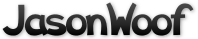Got questions, comments, patches, etc.? Contact Jason Woofenden
diff --git a/draw.h b/draw.h
index d1a2f37..17d2c89 100644 (file)
--- a/draw.h
+++ b/draw.h
@@ -8,24 +8,12 @@ typedef struct _XCol Col;
struct _XFont {
int ascent;
int descent;
-       unsigned int h, w;
+       unsigned int h;
XFontSet set;
XFontStruct *xfont;
};
typedef struct _XFont Fnt;
-/* X11 types - end */
-
-typedef struct {
-       unsigned int w;
-       unsigned int h;
-       int x;
-       int y;
-       int xOff;
-       int yOff;
-} TextExtents;

-
-/* X11 types - begin */
typedef struct _XDraw Draw;
struct _XDraw {
unsigned int w, h;
@@ -39,18 +27,25 @@ struct _XDraw {
Fnt *font;
};

+typedef struct {
+       unsigned int w;
+       unsigned int h;
+       int xOff;
+       int yOff;
+} TextExtents;
+
/* Drawable abstraction */
Draw *draw_create(Display *dpy, int screen, Window win, unsigned int w, unsigned int h);
void draw_resize(Draw *draw, unsigned int w, unsigned int h);
void draw_free(Draw *draw);

/* Fnt abstraction */
-Fnt *font_create(const char *fontname);
-void font_free(Fnt *font);
+Fnt *draw_font_create(Draw *draw, const char *fontname);
+void draw_font_free(Draw *draw, Fnt *font);

/* Colour abstraction */
-Col *col_create(const char *colname);
-void col_free(Col *col);
+Col *draw_col_create(Draw *draw, const char *colname);
+void draw_col_free(Draw *draw, Col *col);

/* Drawing context manipulation */
void draw_setfont(Draw *draw, Fnt *font);
@@ -58,12 +53,12 @@ void draw_setfg(Draw *draw, Col *col);
void draw_setbg(Draw *draw, Col *col);

/* Drawing functions */
-void draw_rect(Draw *draw, int x, int y, unsigned int w, unsigned int h);
-void draw_text(Draw *draw, int x, int y, const char *text);
+void draw_rect(Draw *draw, int x, int y, unsigned int w, unsigned int h, Bool filled, Bool empty, Bool invert);
+void draw_text(Draw *draw, int x, int y, unsigned int w, unsigned int h, const char *text, Bool invert);

/* Map functions */
void draw_map(Draw *draw, int x, int y, unsigned int w, unsigned int h);

/* Text functions */
-void draw_getextents(Draw *draw, const char *text, TextExtents *extents);
+void draw_getextents(Draw *draw, const char *text, unsigned int len, TextExtents *extents);